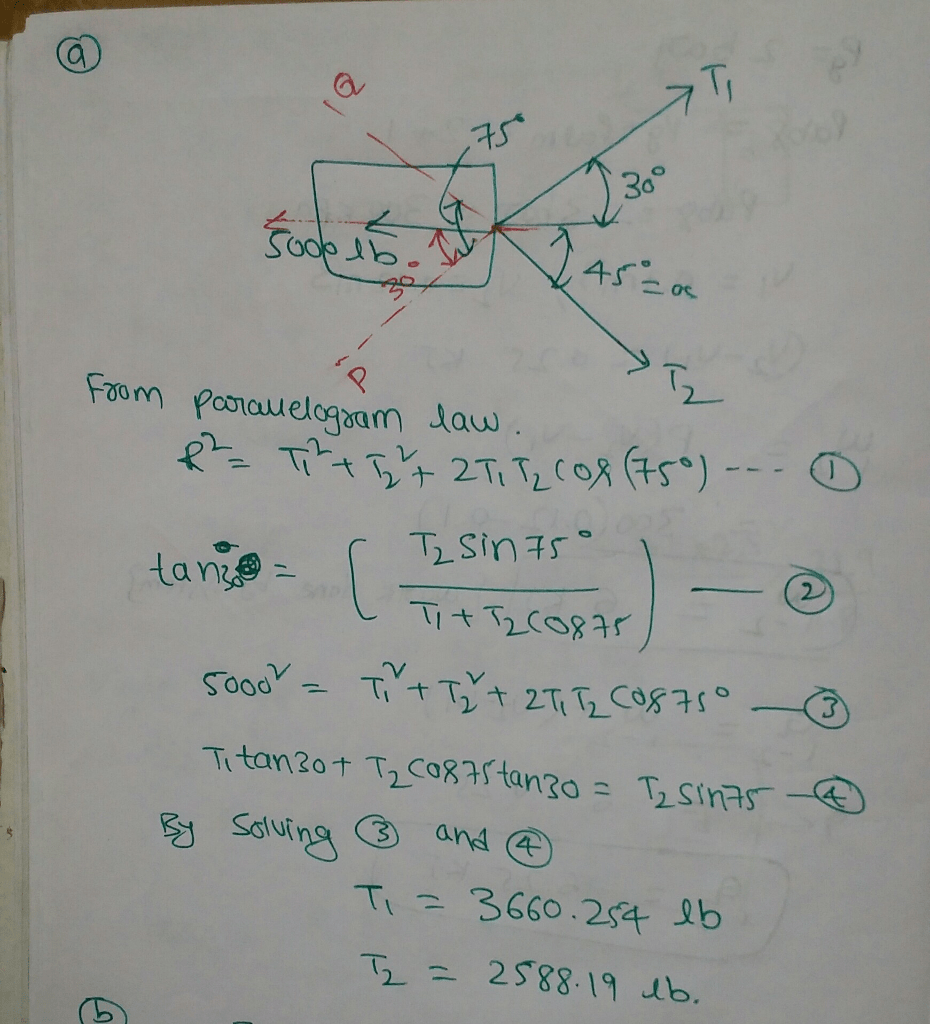# A barge is pulled by two tugboats. If the resultant of the forces excreted by the tugboat is a 5000 lb force directed along the axis of the barge, using the parallelogram law determine: The tension in each of the ropes knowing that alpha = 45 degree the value of alpha for which the tension in rope 2 is minimum.

Question-AnswerCategory: Engineering MechanicsA barge is pulled by two tugboats. If the resultant of the forces excreted by the tugboat is a 5000 lb force directed along the axis of the barge, using the parallelogram law determine: The tension in each of the ropes knowing that alpha = 45 degree the value of alpha for which the tension in rope 2 is minimum.

### A barge is pulled by two tugboats. If the resultant of the forces excreted by the tugboat is a 5000 lb force directed along the axis of the barge, using the parallelogram law determine: The tension in each of the ropes knowing that alpha = 45 degree the value of alpha for which the tension in rope 2 is minimum.